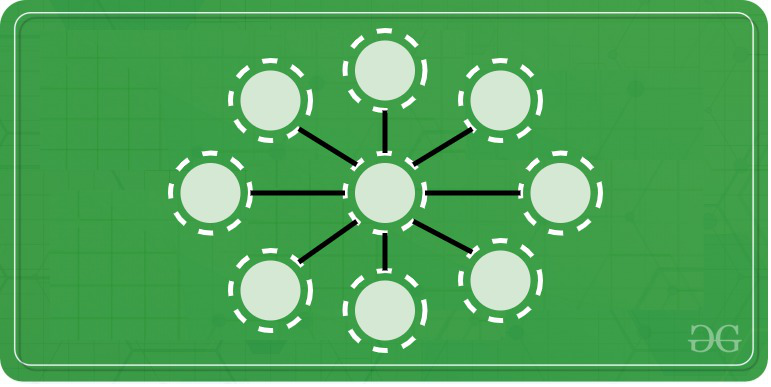Open in App
Not now

# Puzzle | Place numbers 1 to 9 in a Circle such that sum of every triplet in straight line is 15

• Difficulty Level : Basic
• Last Updated : 18 Jan, 2023

Place the numbers 1 to 9 in a circle, so that wherever there are three in a straight line they shall add up to 15.### SOLUTION:

• The sum of first number and last number is ten ( 1 + 9 = 10 ).
• Similarly, the sum of second number and second last element is also ten ( 2 + 8 = 10 )
• In the same way  ( 3 , 7 ) and ( 4 , 6 ) forms two more pairs which add up-to ten.
• So the four pairs ( 1 , 9 ) , ( 2 , 8 ) , ( 3 , 7 ) , ( 4 , 6 ) occupies the external circles or places.
• The inner one is occupied by 5.

1 + 9 + 5 =15

2 + 8 + 5 =15

3 + 7 + 5 =15

4 + 6 + 5 =15

• The pair elements  ( 1 , 9 ) , ( 2 , 8 ) , ( 3 , 7 ) , ( 4 , 6 )  must be placed opposite to each other to attain the required sum. It implies 1 is placed opposite to 9, 2 is placed opposite to 8, 3 is placed opposite to 7 ,4 is placed opposite to 6 and 5 is placed at center.
My Personal Notes arrow_drop_up